## 数据类型

ECSMAScript标准中规定了7种数据类型，这7种数据类型又分为基本型和引用类型。

• `Null`：只包含一个值：`null`
• `Undefined`：只包含一个值：`undefined`
• `Boolean`：包含`true``false`
• `Number`：整数或浮点数，还有一些特殊值（`-Infinity``+Infinity``NaN`
• `String`：字符串
• `Symbol`：表示独一无二的值（ES6加入）

ES10(ES2019)中新增了一种基本类型`BigInt`，可以用来表示超出`number`安全范围的任意精度整数。

• `Object`对象：包含对象、数组、函数等。

## 基本类型和引用类型的区别

### 存放位置不同

• 存储的值大小固定
• 空间较小
• 可以直接操作，效率高

• 存储的值大小不确定，可以动态调整
• 空间较大，运行效率低
• 无法直接操作其内部，使用引用地址读取

``````var a = 10;
var b = 'hello';
var c = true;
``````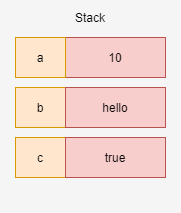``````var a = 10;
var obj1 = { name: 'nihao' };
var obj2 = function () {
// do something
}
``````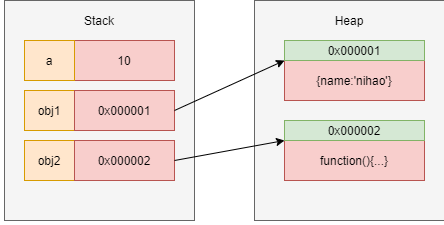### 基本类型具有不可变性

``````var name = 'hello';
name.toUpperCase(); // "HELLO"
console.log(name); // "hello"
``````

``````var c = true;
c = false;
console.log(c); // false
``````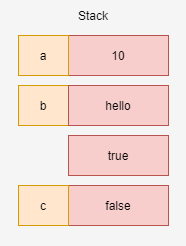``````var obj1 = { name: 'nihao' };
obj1.name = 'nibuhao';
console.log(obj1); // { name: 'nibuhao' }
``````

### 值比较和引用比较

``````var str1 = 'Hello';
var str2 = 'Hello';
console.log(str1 === str2); // true
``````

``````var obj1 = { name: 'obj' };
var obj2 = { name: 'obj' };
console.log(obj1 === obj2); // false
``````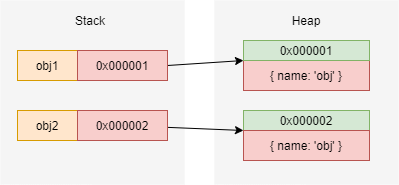### 赋值

``````var str1 = 'Hello';
var str2 = str1;
str2 = 'World';
console.log(str1); // "Hello"
//str1的值没变
``````
``````var obj1 = { name: 'obj1' };
var obj2 = obj1;
obj2.name = 'obj2';
console.log(obj1.name); // "obj2"
// obj1的值改变
``````

## null与undefined

• `null`表示空值
• `undefined`表示“缺少值”，即此处应该有值，但是还未定义
``````var a = null;
console.log(typeof a); // object
console.log(typeof b); // undefined
``````

## Symbol类型

`symbol`变量需要使用`Symbol()`创建

``````var s = Symbol(); // 注意没有new
``````

`Symbol()`函数接受一个可选参数，用来描述即将创建的`symbol`变量，无论传入的描述是否相同，最后生成的`symbol`一定是独一无二的

``````var name1 = Symbol('Tom');
var name2 = Symbol('Tom');
console.log(name1 === name2); // false
``````

``````var name1 = Symbol.for('Tom');
var name2 = Symbol.for('Tom');
console.log(name1 === name2); // true
``````

`Symbol`类型可以用作对象属性，使用该类型可以保证对象不会出现同名属性

``````var obj = {
[Symbol('name')]: 'Tom'
};
``````

``````var obj = {
[Symbol('name')]: 'Tom'
};
for (var key in obj) {
console.log(key); // undefined
}
Object.getOwnPropertySymbols(obj); // [Symbol(name)]
``````

## 数据类型转换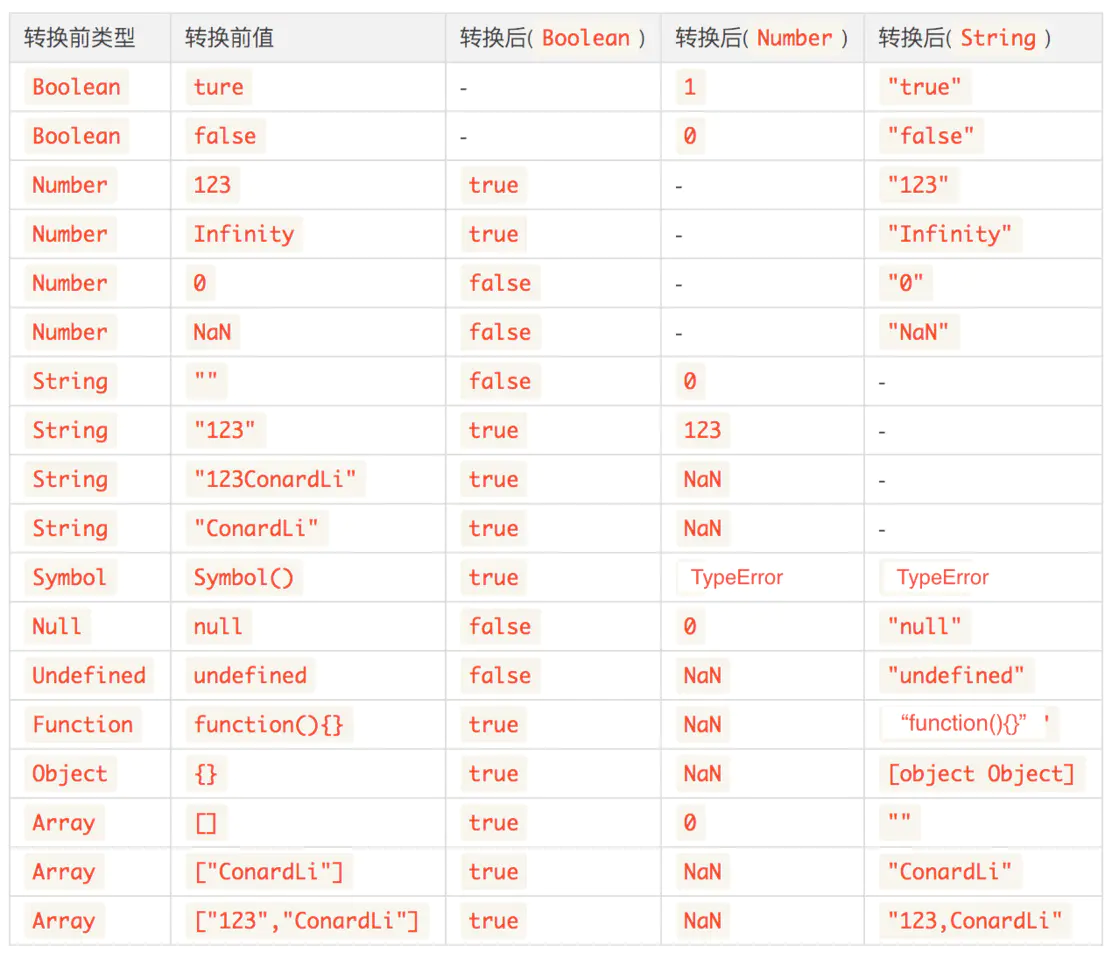### 宽松等号（==）的隐式转换

#### NaN

`NaN`和任何类型比较都会返回`false`，包括他自己

``````NaN == NaN // false
``````

#### Boolean类型与其他类型进行比较

``````false == 0 // true
true == 1 // true
true == 2 // false
``````

``````var x = 10;
if (x) {
// ...
}
if (x == true) {
// ...
}
``````

#### Number类型和String类型进行比较

``````'' == 0 // true
'123' == 123 // true
'1e11' == 1e11 // true
Infinity == 'Infinity' // true
true == '1' // true
false == '0' // true
``````

#### null与undefined

`null == undefined`结果为`true`外，其他任何类型和`null``undefined`比较都为`false`

#### 基本类型与引用类型比较

ToPrimitive规则

• 当引用类型需要被转为基本类型时，它会先查找对象的`valueOf`方法，如果该方法返回基本类型的值，则`ToPrimitive`的结果就是这个值
• 如果`valueOf`不存在或者`valueOf`方法返回的不是基本类型，就会尝试调用对象的`toString`方法，然后使用`toString`的返回值作为`ToPrimitive`的结果
• `valueOf``toString`都不存在，或者没有返回基本类型，则抛出`TypeError`异常

• 引用类型转换为`Number`类型，先调用`valueOf`，再调用`toString`
• 引用类型转换为`String`类型，先调用`toString`，再调用`valueOf`

``````Number([]); // 0
Number(); // 10

var obj = {
valueOf: () => { return 10; },
toString: () => { return -10; }
};
Number(obj); // 10
String(obj); // -10
``````

``````var a = {};
var b = [1, 2, 3];

a == '[object Object]'; // true
a.toString(); // [object Object]
b == '1,2,3' // true
b.toString(); // "1,2,3"
``````

## 判断数据类型

### typeof

`typeof`只能用来判断以下几个类型

``````typeof 'str';  // string
typeof 123;  // number
typeof true;  // boolean
typeof Symbol();  // symbol
typeof undefined;  // undefined
typeof function () {} // function
``````

### instanceof

`instanceof`可以判断引用类型的具体类型

``````[] instanceof Array; // true
/1/ instanceof RegExp; // true
new Date() instanceof Date; // true
``````

``````null instanceof null; // Uncaught TypeError: Right-hand side of 'instanceof' is not an object
null instanceof Object; // false
``````

MDN中，`instanceof`被这样描述：

`instanceof` 运算符用于检测构造函数的 `prototype` 属性是否出现在某个实例对象的原型链上。

### Object.prototype.toString.call()

`Object.prototype.toString.call()`利用`call`来改变`this`的指向，可以让该方法能够获取到任意变量的`[[class]]`属性，通过该属性可以判断所有`JavaScript`的内置类型

``````Object.prototype.toString.call(null); // [object Null]
Object.prototype.toString.call(undefined); // [object Undefined]
Object.prototype.toString.call(123); // [object Number]
Object.prototype.toString.call(new Date()); // [object Date]
// ...
``````

``````function Person() {}
Object.prototype.toString.call(new Person()); // [object Object]
new Person() instanceof Person; // true
``````

## 参考资料

• 发表于 2020-07-11 12:00
• 阅读 ( 97 )
• 分类：网络文章

## 作家榜 »

1.小编 文章